# How to Find Variance in Excel

Variance is a powerful data analysis tool used to calculate how much individual results vary from the average value. It’s usually used for numerical data in Excel, but it can also measure various other types of information. Calculating variance in Excel might seem complicated at first, but once you figure out what each function is used for, you’ll be able to do it in seconds.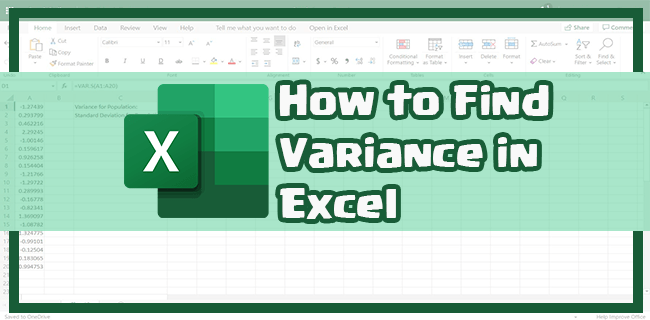This article will teach you how to find variance in Excel on a Windows PC, Mac, and iPad. We’ll also explain what each function is used for and how they can help you calculate and estimate various types of data.

## How to Find Variance in Excel on a Windows PC

To calculate variance in Excel means to measure how far different values are from the average value (or the mean). In Excel, finding variance usually refers to calculating a set of numbers in a data range against their average value.

In simple terms, we use variance in Excel to determine to what extent individual results vary from the average result. The more the numbers grow, the greater the variance. However, if the variance equals zero, all the numbers from the data set are the same.

In Excel, the variance tool can be used to calculate various metrics. For example, the age group of a population, exam results, expenses, and more.

There are different types of variance functions in Excel, depending on the type of variance and the size of your data set. There are six basic variance functions you can use for sample variance and population variance calculations. These include VAR, VAR.P, VARP, VAR.S, VARA, and VARPA functions. There are other functions available in Excel, but we won’t need them for this guide.

Before we go into how to find variance in Excel, you need to figure out which variance function to use. If you have a smaller data set, you can use VAR, VARA, and VAR.S functions. For a larger sample, the best variance functions are VAR.P, VARP, and VARPA.

Sample variance is used if your spreadsheet entails only a sample of the population, whereas if the entire population is estimated, you’ll need to use the population variance. The VAR.P function is used to calculate the variance based on the entire population, and it ignores logical values and text. The VARA function also measures the variance based on the entire population, but it doesn’t ignore logical values and text. In contrast, the VAR.S function estimates the variance based on one sample from the population.

The type of variance function you should use depends on which version of Excel you have. For example, the VAR.S function is the newer version of the VAR function. The same goes for the VAR.P function, which is used instead of the VARP function in newer versions of Excel.

Let’s say you want to calculate a sample variance in Excel. You want to find the variance for your students’ exam scores, where column A is for the students’ names, and column B is for their scores.

1. Launch Excel on your Windows PC.2. Open the spreadsheet where you want to find variance. If you want to create a new spreadsheet, select “Blank workbook.”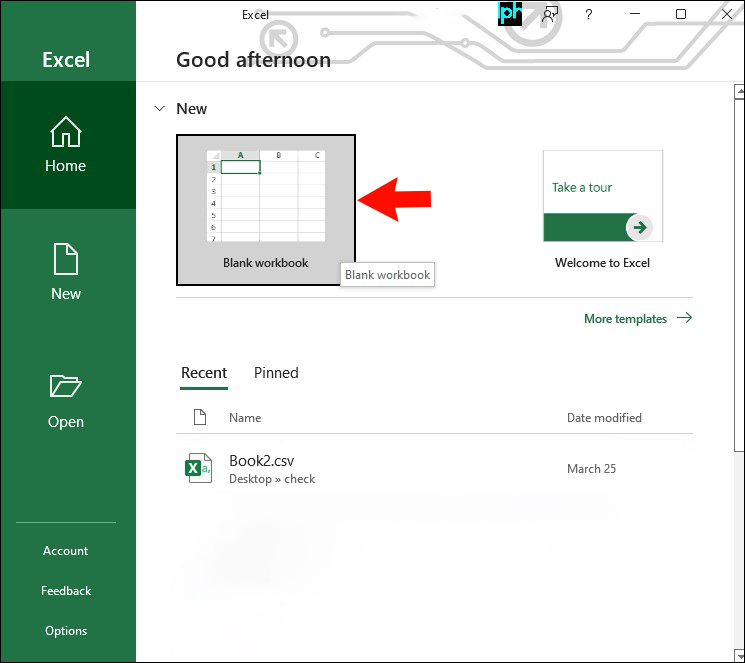3. Enter any the necessary data in the spreadsheet.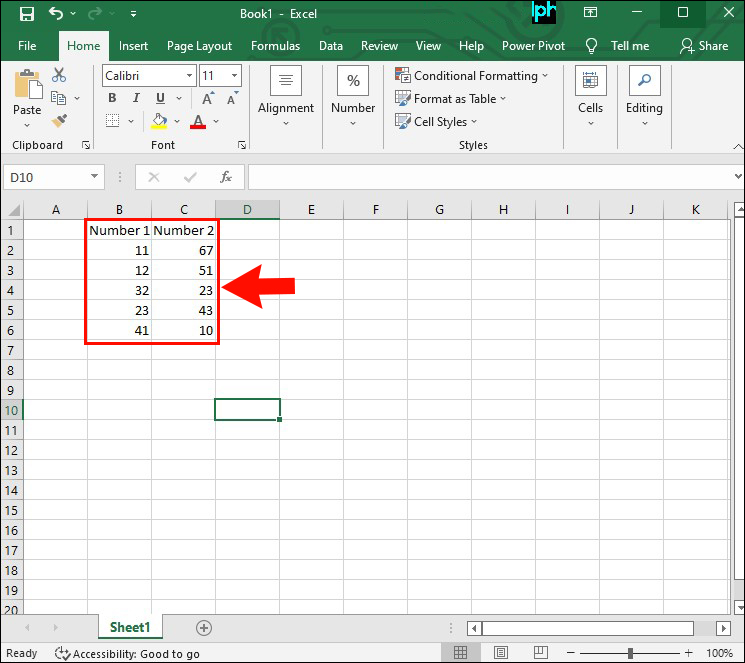Note: Make sure all the data is written in a range (a column or a row) or you won’t be able to find variance.5. Type in “`=VAR” (“=VAR.S`” if you have the newest version of Excel) in the cell.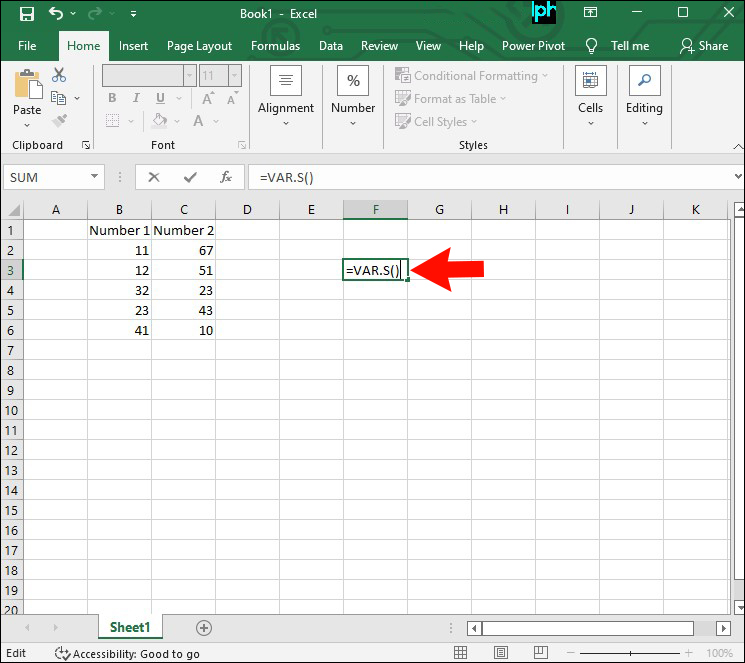6. In parentheses, write the range. For example: “(B2:B11)”.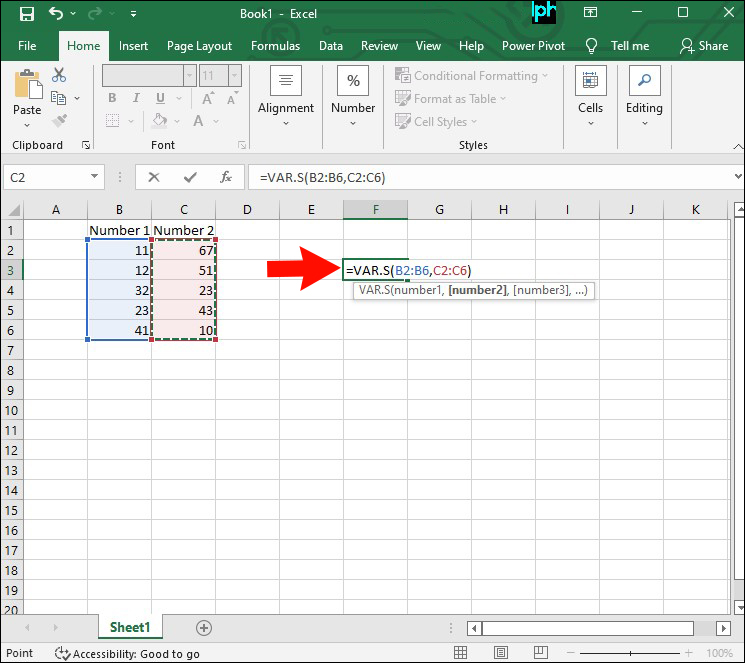7. Press the “Enter” key.The variance will immediately appear in the same cell where you wrote the function.

If you want to calculate a population variance, you’ll be using the VARP or the VAR.P function, depending on which version of Excel you have. A population variance indicates that the data in your spreadsheet represents the entire population of something. Here’s how it’s done.2. Double-click on an empty cell.3. Type in “`=VARP`” or “`=VAR.P`” for newer Excel versions.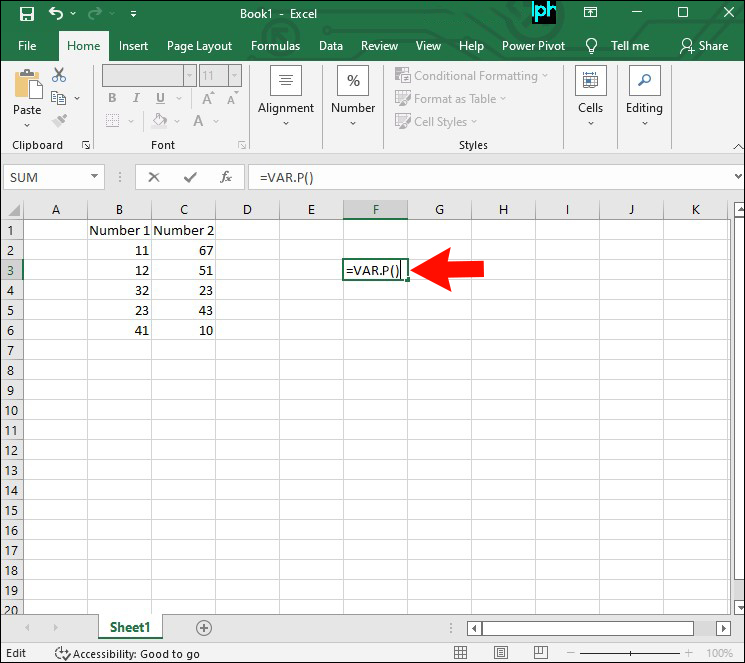4. Enter the range in parentheses, like in this example “(A2:A15)”.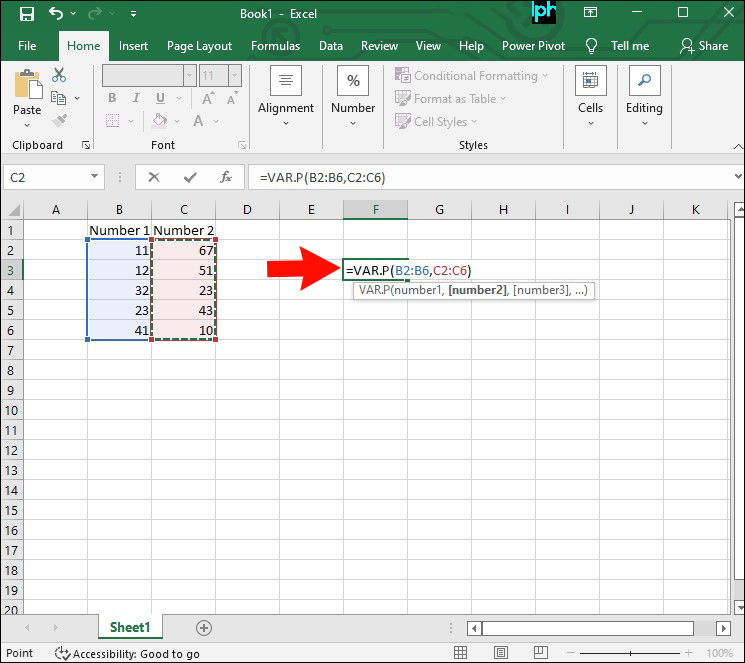5. Press “Enter.”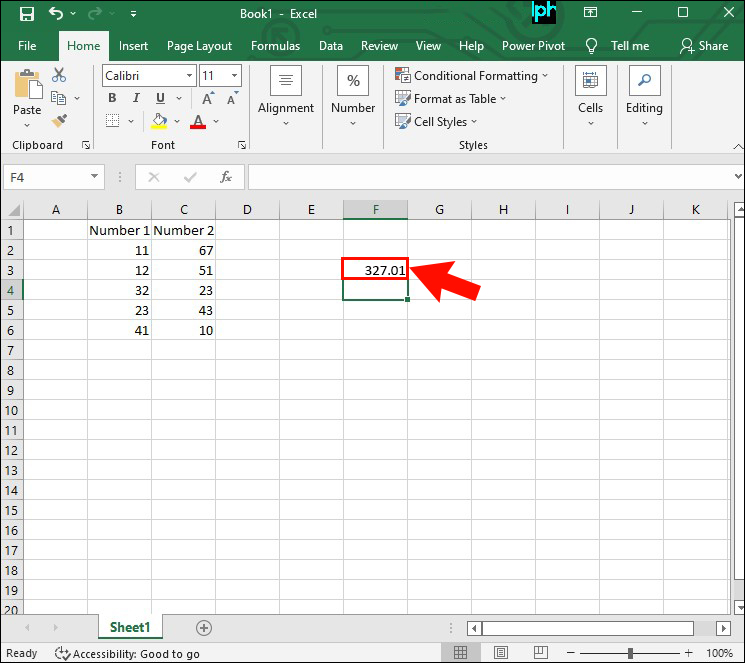The population variance will appear in that same cell.

## How to Find Variance in Excel on a Mac

If you have a Mac, you can use it to find variance in Excel. If you want to calculate a sample variance for a data set, follow the steps below.

1. Run Excel on your Mac.
2. Open an old spreadsheet or create a new one.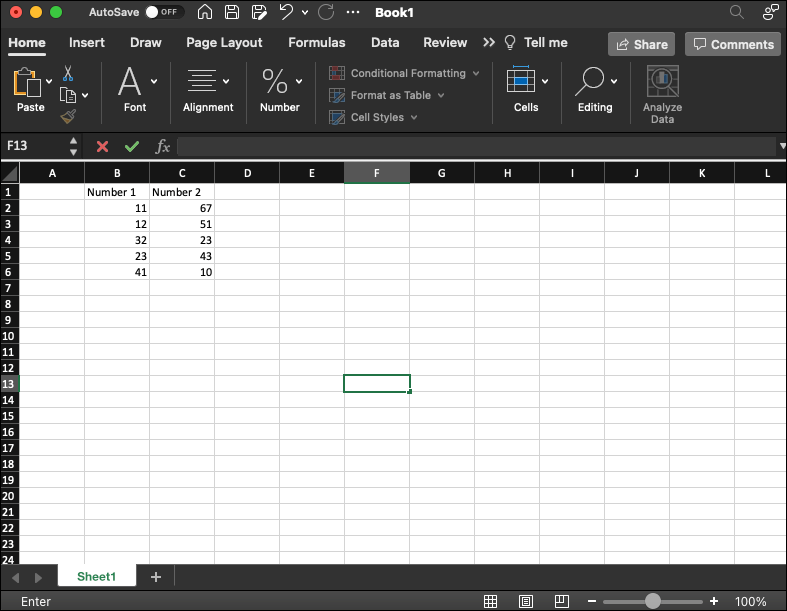3. Make sure the data is entered in the same range.4. Choose an empty cell anywhere on your spreadsheet and double-click on it.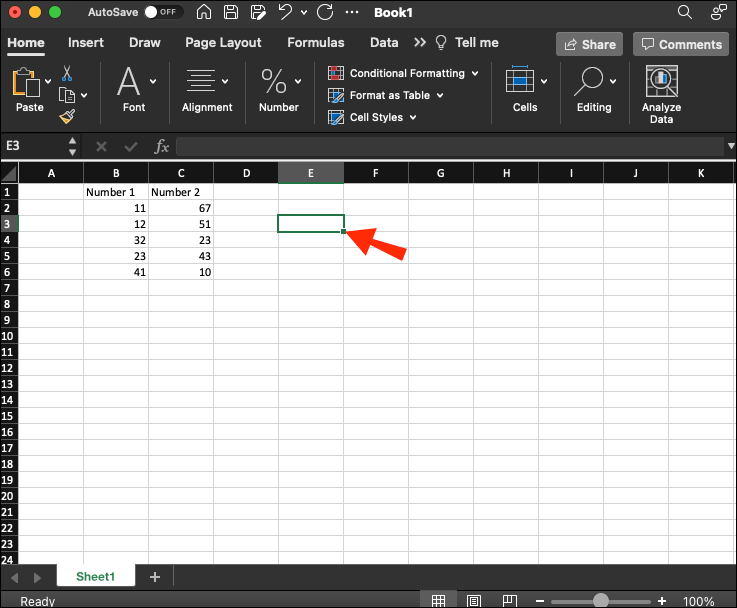5. Enter the sample variance function “`=VAR`” or “`=VAR.S`”.6. Type in the range in parentheses. For example: “`(A4:A39)`”.Note: Make sure there isn’t any space between the function and the range. It’s supposed to look like this: “`=VAR.S(A4:A39)`”.
7. Press the “Enter” key.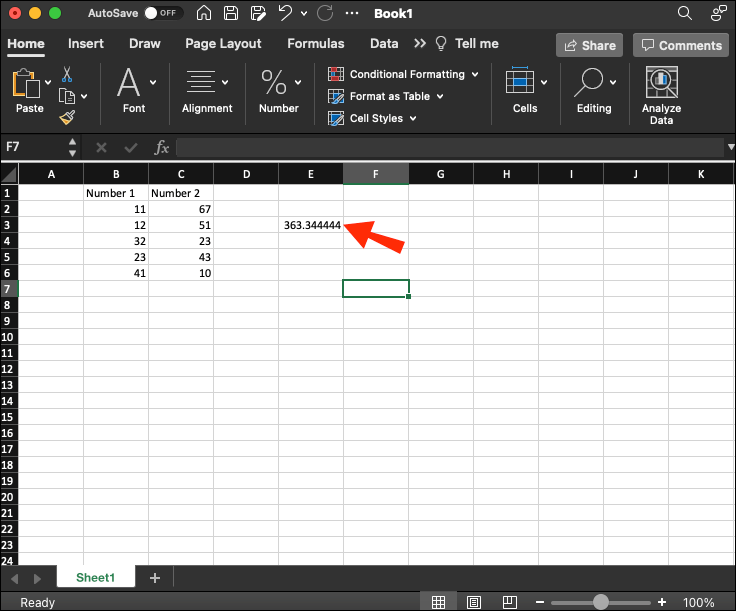That’s all there is to it. The sample variance will immediately be calculated in the same cell where you entered the function. If you’re working with a larger population, use the VAR.P function. Follow the steps below to find out how it’s done.

2. Double-click on an empty cell and enter “`=VAR.P`”.3. Write the range in parenthesis, like in this example: “`=VAR.P(B2:B50)`”.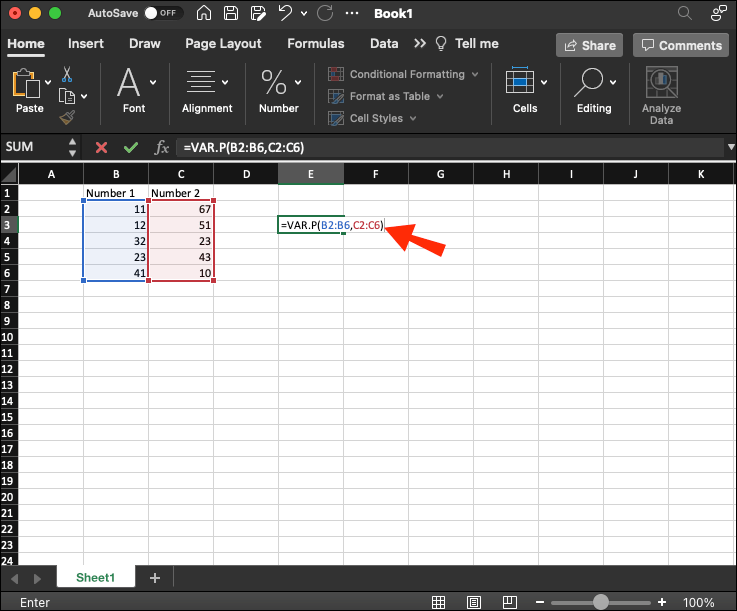4. Press “Enter” on your keyboard.## How to Find Variance in Excel on an iPad

If you don’t have your laptop with you at the moment, you can use your iPad to find variance in Excel. The process is mainly similar, you’ll just be working on a smaller screen. Here’s what you need to do to calculate variance in Excel on an iPad.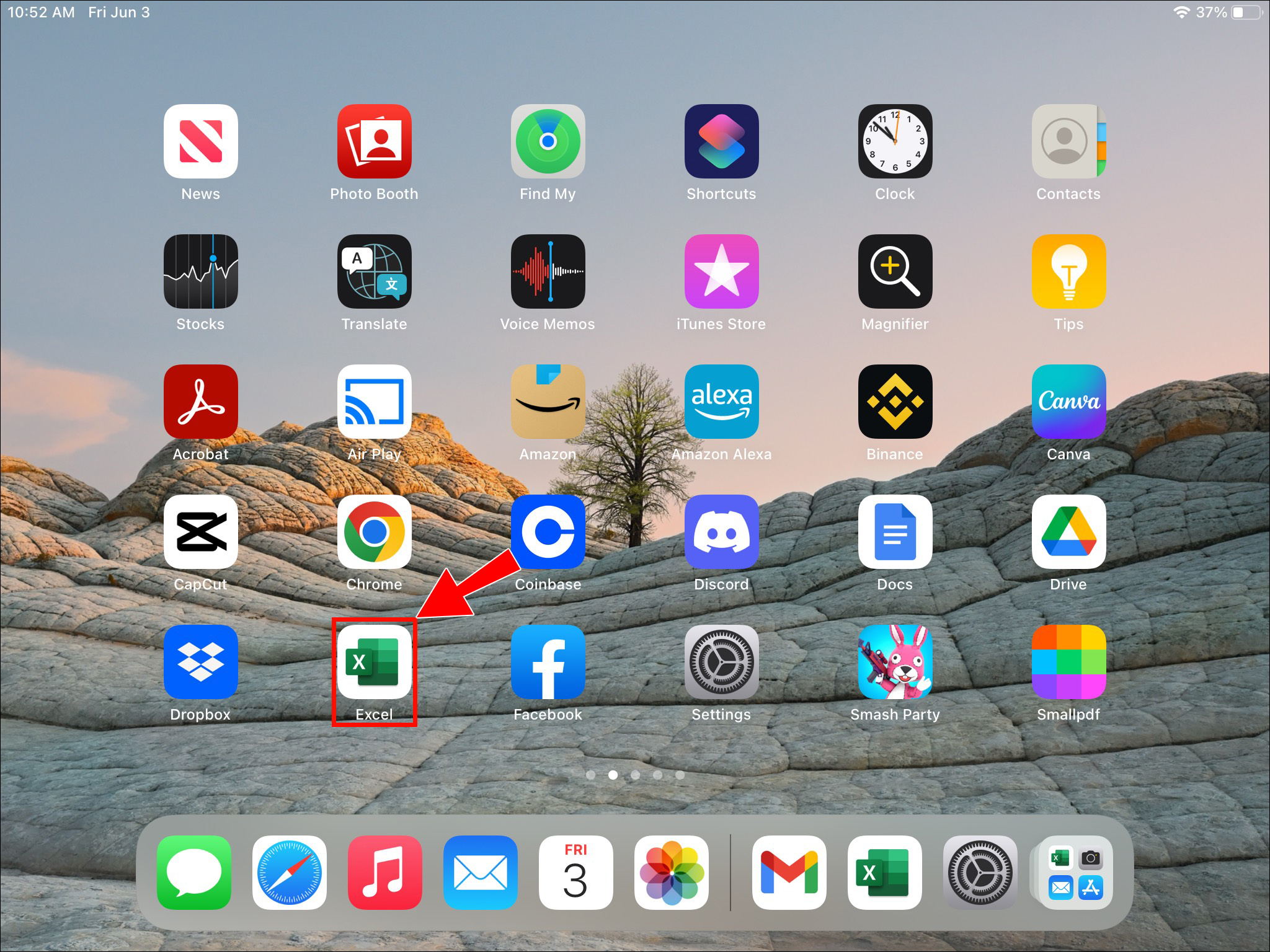2. Choose a spreadsheet from the “New” or “Recent” sections.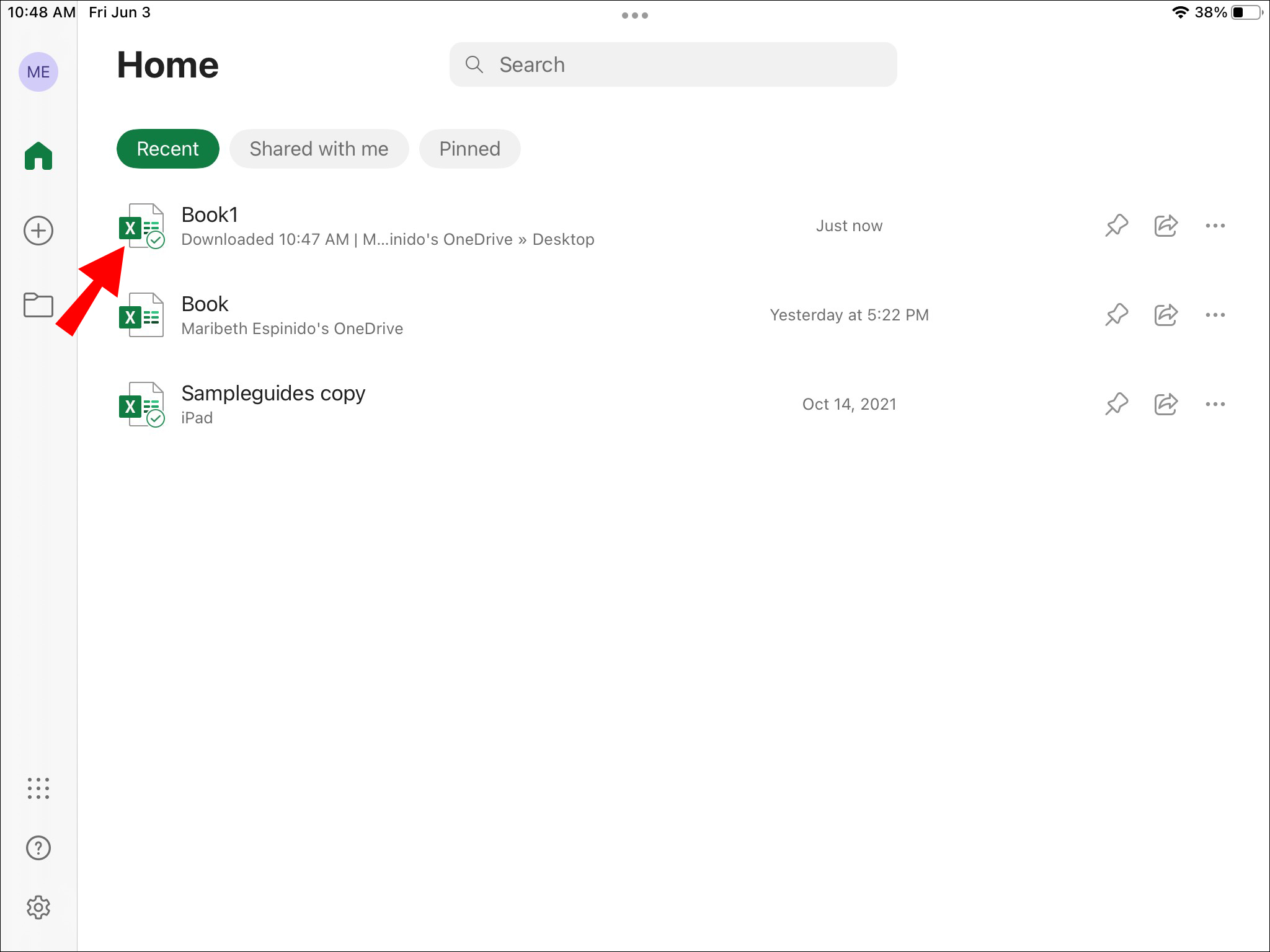3. Tap on an empty cell to select it.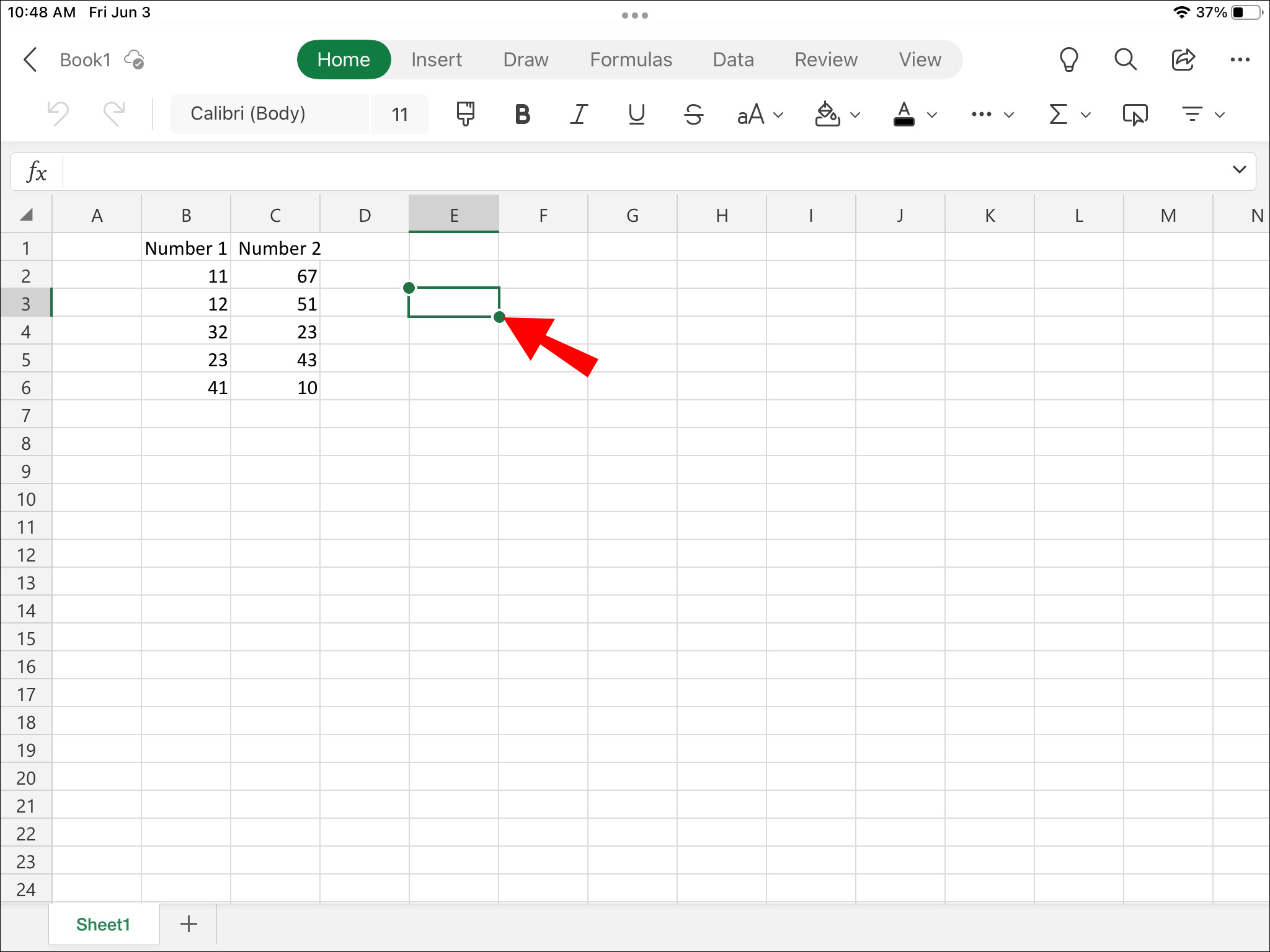4. Enter the variance function in the “fx” bar above the spreadsheet. For example: “`=VAR.P(A3:A15)`”.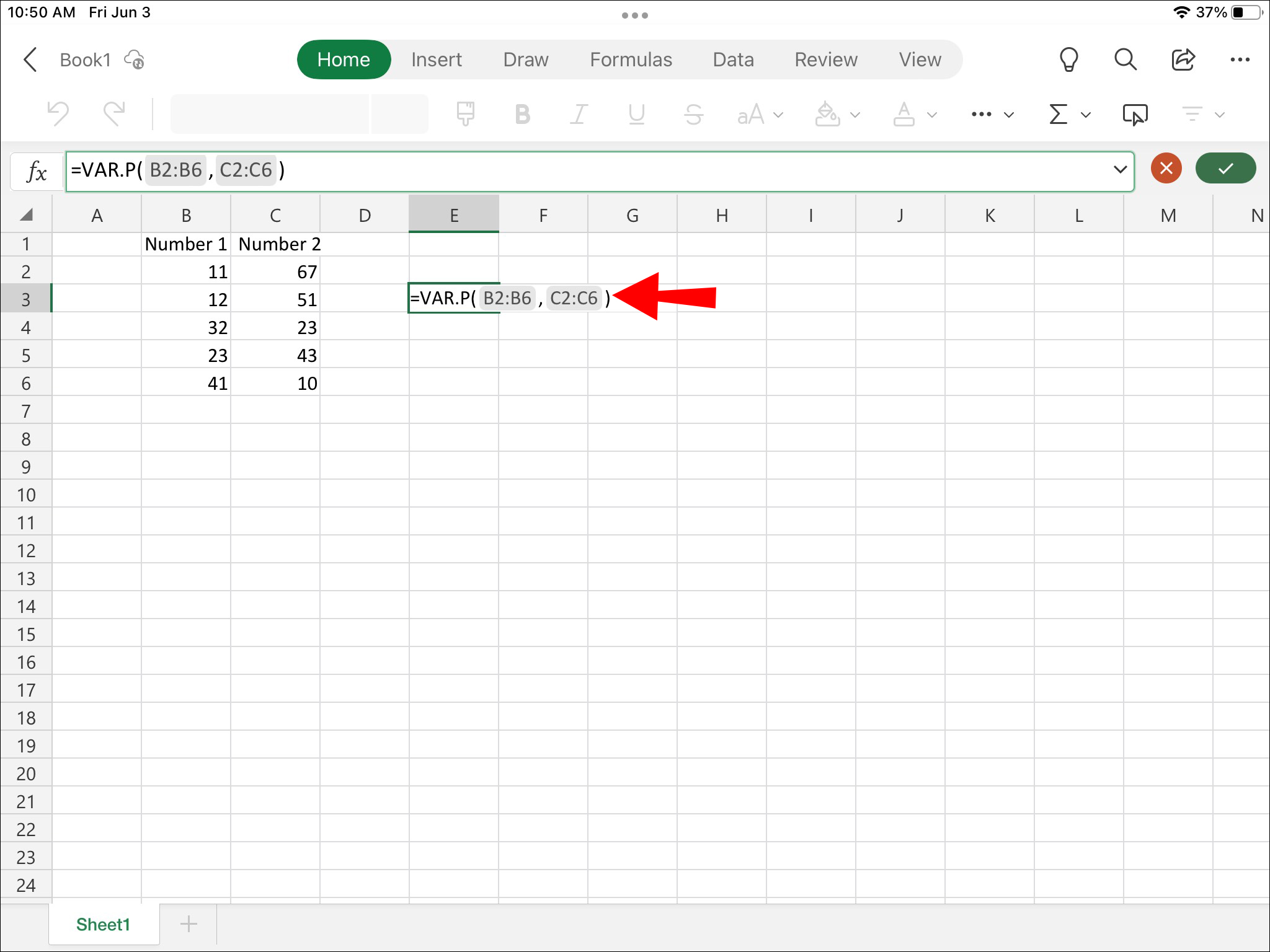The variance will automatically appear in the cell you had previously selected.

No matter which device you use to find variance in Excel, it will only take you a few minutes to get it done. You just need to figure out which variance function works best with your data set, and the rest is easy. You’ll be able to estimate different types of variances, whether they refer to numerical or logical data.

Have you ever calculated variance in Excel before? Which variance function did you use to do this? Let us know in the comments section below.

Disclaimer: Some pages on this site may include an affiliate link. This does not effect our editorial in any way.

Todays Highlights